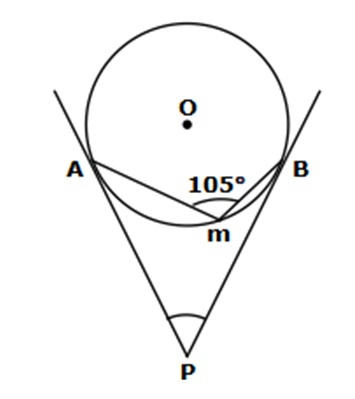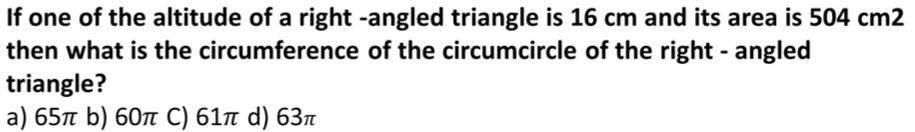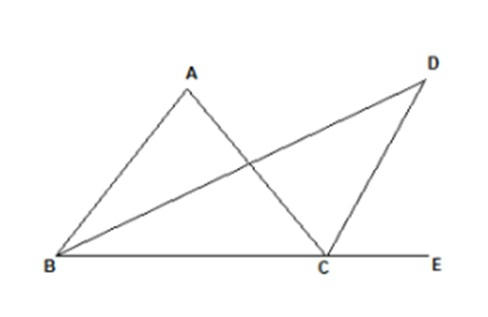# SSC Mock Set – 1 | Maths & Reasoning | Free PDF Download

1. If (3x2 – 11x + p) and (4x2 – 15x + q) both are divisible by (x – 3), then which of the following is true relationship between p and q.

a) pq = 54    b) q/p = 2    c) p+q=10      d) q – p = 2

2. Find the value of angle APB in the following figure. O is the centre of the circle and PA and PB are tangents to the circle.

a) 45°       b) 60°         c) 15°         d) 30°3.4. What is the area of a triangle inscribed in a square of side 8 cm in such a way that the base of the triangle is on one side of the square and vertex of the triangle lies at opposite side of the square?
a) 64 cm2     b) 16 cm2      c) 24 cm2    d) 32cm2

5.In an isosceles triangle ABC, the sum of similar angle of the triangle is half of the third angle of that triangle. Length of similar sides of the triangle is 8 cm. Find the length of circumradius of the triangle in cm
a) 12     b) 16       c) 4    d) 12

6.Five years ago, the average age of a family of four members was 20 years. At present, a new member, i.e. son-in-law has joined the family and the son has left after marriage. Now, the sum of ages of all the members of the family is 92 years. Find the difference between age of son and son-in-law (in years).
a) 6     b) 8      c) 9    d) 7

6.The volumes of a sphere and a right circular cylinder having the same radius are equal. The ratio of the diameter of the sphere to that of the height of the cylinder is
a) 3 : 2     b) 2 : 3      c) 1 : 2    d) 1 : 3

7.What least value should be added to 2784 to make it divisible by 12, 13 and 18?
a) 444     b) 24      c) 468    d) 38

8.If p, q and r are three positive numbers, such that p + q = 10, q + r = 12 and
p
+ r = 8, what will be the average of p, q and r?
a) 7     b) 15     c) 5     d) 10

9.What is the unit digit of 4744+8221-5369?
a) 6     b) 0     c) 3     d) 2

10.In the given figure, bisectors of angle ABC and angle ACE are produced to intersect at point D. Angle DCE measures 65°. If angle BDC measures 35°, find the measure of angle ABD.
a) 45°      b) 30°      c) 60°      d) 15°